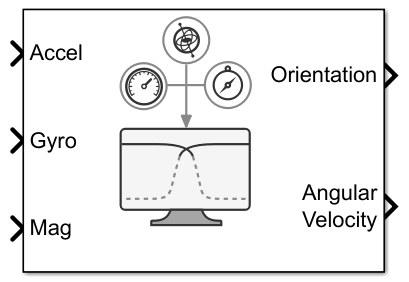# Complementary Filter

Estimate orientation using complementary filter

Since R2023a

•Libraries:
Sensor Fusion and Tracking Toolbox / Multisensor Positioning / Navigation Filters

## Description

The Complementary Filter Simulink® block fuses accelerometer, magnetometer, and gyroscope sensor data to estimate device orientation.

## Ports

### Input

expand all

Accelerometer readings in the sensor body coordinate system in m/s2, specified as an N-by-3 matrix of real numbers. N is the number of samples, and the each row is of the form [x y z].

Data Types: `single` | `double`

Gyroscope readings in the sensor body coordinate system in rad/s, specified as an N-by-3 matrix of real numbers. N is the number of samples, and the each row is of the form [x y z].

Data Types: `single` | `double`

Magnetometer readings in the sensor body coordinate system in µT, specified as an N-by-3 matrix of real numbers. N is the number of samples, and the each row is of the form [x y z].

#### Dependencies

To enable this input port, select the ```Enable Magnetometer input``` parameter.

Data Types: `single` | `double`

### Output

expand all

Orientation of the sensor body frame relative to the navigation frame, returned as an M-by-4 matrix of real numbers or a 3-by-3-by-M array. Each row of the M-by-4 matrix represents the four components of a `quaternion`. Each page of the 3-by-3-by-M array represents a 3-by-3 rotation matrix.

The number of input samples, N, determines the output size, M.

The output format depends on the value of the `Orientation format` parameter.

Data Types: `single` | `double`

Angular velocity, with gyroscope bias removed, in the sensor body coordinate system in rad/s, returned as an M-by-3 matrix of real numbers.

The number of input samples, N, determines the output size, M.

Data Types: `single` | `double`

## Parameters

expand all

Specify the navigation reference frame as `NED` (North-East-Down) or `ENU` (East-North-Up).

Specify the format in which to output `Orientation` as `quaternion` or ```Rotation matrix```:

• `quaternion``Orientation` outputs an M-by-4 matrix of real numbers. Each row of the matrix represents the four components of a `quaternion`.

• `Rotation matrix``Orientation` outputs a 3-by-3-by-M array, in which each page of the array is a 3-by-3 rotation matrix.

The number of input samples, N, determines the output size, M.

Specify the accelerometer gain as a real scalar in the range [0, 1]. The gain determines how much the block trust the accelerometer measurement over the gyroscope measurement for orientation estimation.

Example: `0.02`

Data Types: `single` | `double`

Select this parameter to enable input of magnetometer readings at the `Mag` port.

Specify the magnetometer gain as a real scalar in the range [0, 1]. The gain determines how much the block trust the magnetometer measurement over the gyroscope measurement for orientation estimation.

Example: `0.02`

Data Types: `single` | `double`

Select the type of simulation to run from these options:

• `Interpreted execution` — Simulate the model using the MATLAB® interpreter. This option shortens startup time, but has a slower simulation speed than `Code generation`. In this mode, you can debug the source code of the block.

• `Code generation` — Simulate the model using generated C code. The first time you run a simulation in this mode, Simulink generates C code for the block. Simulink reuses the C code for subsequent simulations, as long as the model does not change. This option requires additional startup time, but the speed of subsequent simulations is comparable to ```Interpreted execution```.

## Version History

Introduced in R2023a